# GED Math : Operations with Negative Numbers

## Example Questions

### Example Question #31 : Operations With Negative Numbers

Evaluate: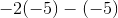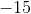Explanation:

Use the order of operations to evaluate this.

When there are double negatives, the value will be converted to a positive.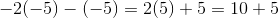When there is no sign in between an integer and the parentheses, it will be multiplied.

The answer is:### Example Question #31 : Operations With Negative Numbers

Solve: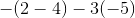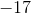Explanation:

Evaluate the terms in the parentheses first.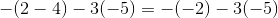Double negatives will be converted to a positive.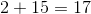The answer is:### Example Question #31 : Operations With Negative Numbers

Evaluate: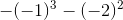Explanation:

Evaluate using order of operations.  Expand the terms in parentheses and exponents first before distributing the negative signs.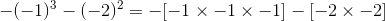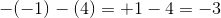The answer is:### Example Question #34 : Operations With Negative Numbers

Evaluate: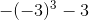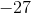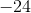Explanation:

Expand the term with the power of three.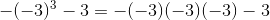Expand the terms.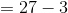The answer is:### Example Question #35 : Operations With Negative Numbers

Evaluate: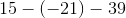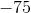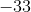Explanation:

Double negatives will convert to a positive.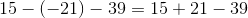Determine the sum.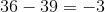The answer is:### Example Question #36 : Operations With Negative Numbers

Evaluate: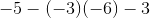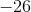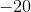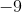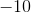Explanation:

Use order of operations to solve.  Evaluate the terms in parentheses first.

Double negatives will turn into a positive.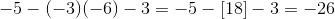The answer is:### Example Question #151 : Basic Operations

Evaluate: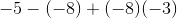Explanation:

Evaluate using order of operations first.  Double negatives will turn into a positive.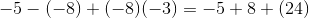Determine the sum.

The answer is:### Example Question #152 : Basic Operations

Solve: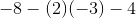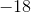Explanation:

Expand the expression by order of operations.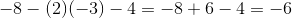The answer is:### Example Question #31 : Operations With Negative Numbers

Solve: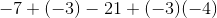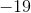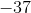Explanation:

Solve by first evaluating the parentheses.  Double negatives result in a positive sign, while a positive and negative sign will result in a negative sign.

Simplify the terms so that there is one sign remaining for each integer.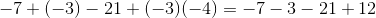Evaluate the sum.

The answer is:### Example Question #154 : Basic Operations

Evaluate: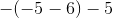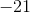Explanation:

Evaluate the terms of the inner parentheses.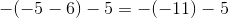Double negatives translates to a positive sign.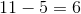The answer is:### All GED Math Resources